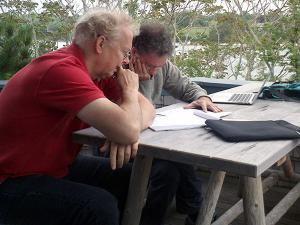# Theoretical Frameworks for Intelligence

### Research Thrust: Theoretical Frameworks for IntelligenceUnderstanding intelligence and the brain requires theories at different levels, from the biophysics of single neurons, to algorithms and circuits, to overall computations and behavior, to a theory of learning. Modeling work draws from advances in statistical learning theory, machine learning, probabilistic inference, and the biophysics of computation. Many computational methods used in these areas are described in the lectures for the other research thrusts. The lectures here provide a broad introduction to machine learning methods, including statistical learning theory. Supporting materials for the lectures and MATLAB activities presented by Lorenzo Rosasco can be found at http://lcsl.mit.edu/courses/cbmmss/.

### Tomaso Poggio: Learning as the Prototypical Inverse Problem

Topics: Overview of learning tasks and methods, ill-posedness and regularization, basic concepts and notation; supervised learning: given training set of labeled examples drawn from a probability distribution, find a function that predicts the outputs from the inputs; noise and sampling issues; goal is to make predictions about future data (generalization); loss function measures the error between actual and predicted values; examples of loss functions for regression and binary classification; expected risk measures loss averaged over unknown distribution; empirical risk as proxy for expected risk; hypothesis space of functions or models to search (e.g. linear functions, polynomial, RBFs, Sobolev spaces); minimizing empirical risk; learning algorithm should generalize and be well-posed, e.g. stable; regularization: classical way to restore well-posedness and ensure generalization; Tikhonov regularization; intelligent behavior optimises under constraints that are critical to problem solving and generalization

### L. Mahadevan: Ill Posed Problems

Topics: Inverse problems are pervasive in physics, geophysics, biology, engineering; definition of direct vs. inverse problem; direct problem may involve linear or nonlinear operator, and there may be noise; definition of well-posed problems (solution exists and is unique and stable); issues of sampling, noise, stability; inverse problems are typically ill-posed and can be regularized, for example, by minimizing both accuracy and smoothness; linear (matrix) inverse problems, SVD, least squares solution, eliminating infeasible solutions, selecting relative weight of accuracy vs. smoothness terms; example: blurring/deblurring using Tikhonov regularization; probabilistic (Bayesian) approach to inverse problems, e.g. MLE and MAP; minimizing least squares error for linear inverse problems is analogous to maximizing the likelihood if noise is Gaussian

### Lorenzo Rosasco: Learning Theory, Part 1 (local methods, bias-variance, cross validation) and Part 2 (regularization: linear least squares, kernel least squares)

Topics: Supervised learning, nearest neighbor methods and overfitting, k-nearest neighbors - choosing k, bias-variance tradeoff, cross validation, regularization, least squares, linear systems, computational complexity, kernel least squares using linear, polynomial, and Gaussian kernels

### Lorenzo Rosasco: Learning Theory, Part 3 (variable selection (OMP), dimensionality reduction (PCA))

Topics: Determining which variables are important for prediction (e.g. given n patients and p genes, which genes are most important for prediction), sparsity (only some coefficients are non-zero), brute force, greedy approaches/matching pursuit, basis pursuit/lasso, unsupervised learning, dimensionality reduction, PCA